Trusted answers to developer questions
Trusted Answers to Developer Questions

Related Tags

calculus
community creator
deep learning
chain rule

# Chain Rule in Matrix Calculus for Deep Learning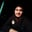Kiran U Kamath

Grokking Modern System Design Interview for Engineers & Managers

Ace your System Design Interview and take your career to the next level. Learn to handle the design of applications like Netflix, Quora, Facebook, Uber, and many more in a 45-min interview. Learn the RESHADED framework for architecting web-scale applications by determining requirements, constraints, and assumptions before diving into a step-by-step design process.We can’t compute partial derivatives of very complicated functions using just the basic matrix calculus rules we’ve seen in part 1. For example, we can’t take the derivative of nested expressions, like sum(w + x), directly without reducing it to its scalar equivalent. We need to be able to combine our basic vector rules using the vector chain rule.

In the paper, “The Matrix Calculus You Need For Deep Learning” the authors have defined and named three different chain rules:

1. single-variable chain rule
2. single-variable total-derivative chain rule
3. vector chain rule

The chain rule comes into play when we need the derivative of an expression composed of nested subexpressions. It helps to solve problems by breaking complicated expressions into subexpression whose derivatives are easy to compute.

## Single-variable chain rule

Chain rules are defined in terms of nested functions, like y=f(g(x)) for a single variable chain rule.

The formula is:

dy/dx = (dy/du) (du/dx)

There are 4 steps you use to solve using the single variable chain rule:

1. Introduce intermediate variable.
2. Compute derivatives of intermediate variables w.r.t.(with respect to) their parameters.
3. Combine all the derivatives by multiplying them together.
4. Substitute variables back in the derivative equation.

Let’s see some examples of a nested equation

y = f (x) = ln(sin(x³ ) ² ):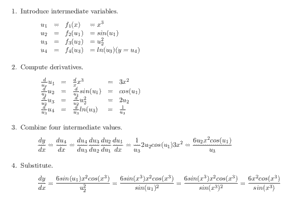You can use the nested equation to compute the derivatives of the intermediate variables in isolation!

However, the single variable chain rule is only applicable when a single variable can only influence the output in one way. As you can see in the example, we can handle nested expression of a single variable (x) using this chain rule, but only when x can affect y through a single data flow path.

## Single-variable total-derivative chain rule

If we apply a single variable chain rule to y = f (x) = x + x² we will get the wrong answer because the derivative operator does not apply to multivariate functions. If you change x in the equation, it affects y both as the operand of addition and as the operand of square. We clearly can’t apply the single variable chain rule here; so, we move to total derivatives, which is to compute (dy/dx). Now, we need to sum up all possible contributions from changes in x to the change in y.

The formula for the total derivative chain rule is: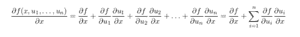Total derivative assumes that all variables are potentially co-dependent whereas partial derivative assumes that all variables but x are constants.

When you take the total derivative with respect to x, other variables might also be functions of x; so, you should add in their contributions as well. The left side of the equation looks like a typical partial derivative, but the right-hand side is the total derivative.

Lets see an example: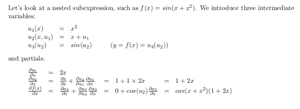The total derivative formula always sums up terms in the derivative. For example, given y = x × x² instead of y = x + x² , the total-derivative chain rule formula still adds partial derivative terms, for more detail see a demonstration here.

The total derivative formula can be simplified further: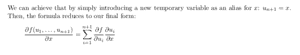This chain rule takes into consideration the total derivative degenerates to the single-variable chain rule when all intermediate variables are functions of a single variable.

## Vector chain rule

The derivative of a sample vector with respect to a scalar is y = f (x):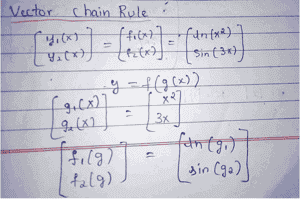Introduce two intermediate variables, g 1 and g 2 , one for each f i so that y looks more like y = f (g(x)):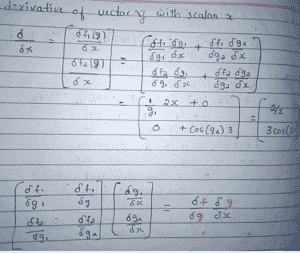If we split the terms, isolating the terms into a vector, we get a matrix by vector: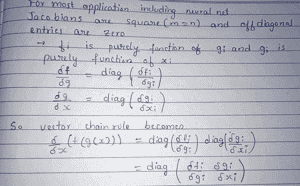The vector chain rule is the general form as it degenerates to the others. When f is a function of a single variable x and all intermediate variables u are functions of a single variable, the single-variable chain rule applies. When some or all of the intermediate variables are functions of multiple variables, the single-variable total-derivative chain rule applies. In all the other cases, the vector chain rule applies.

This completes the chain rule. In next shot, part 3, we will see how we can apply this gradient of neural activation and loss function and wrap up.

Thank you.

This is part 2, in part 3 I will explain the gradient of neuron activation.

RELATED TAGS

calculus
community creator
deep learning
chain rule

CONTRIBUTORKiran U Kamath